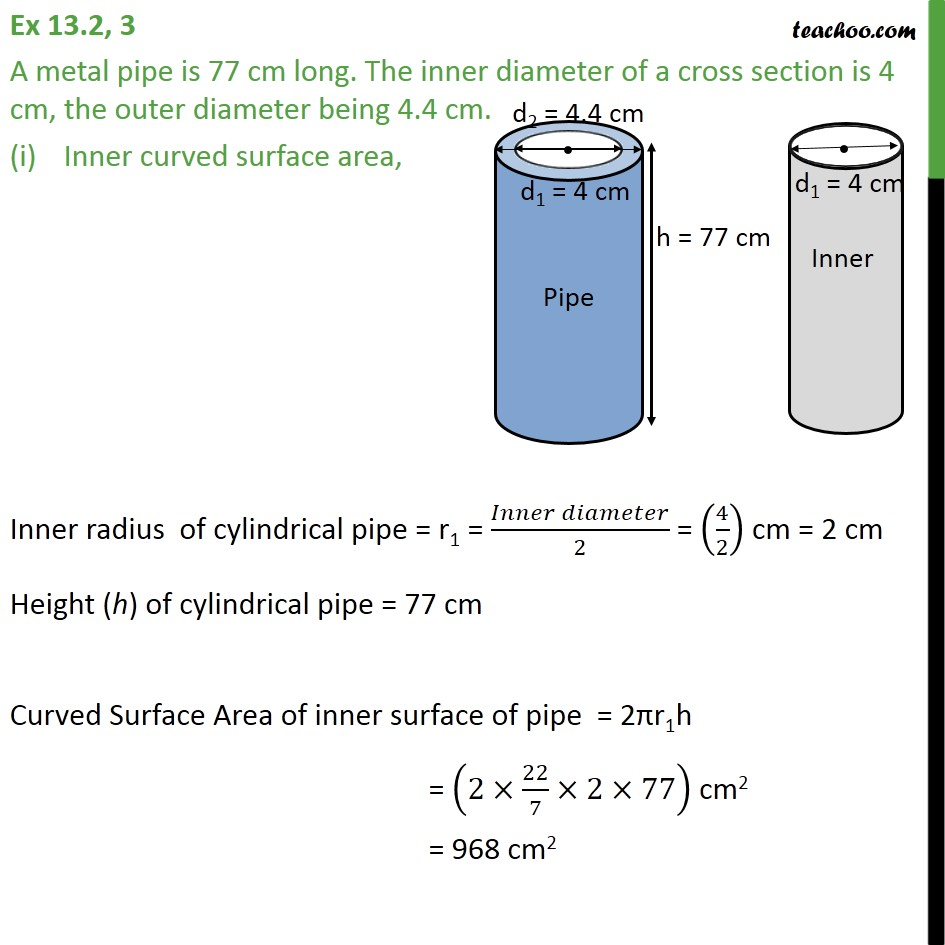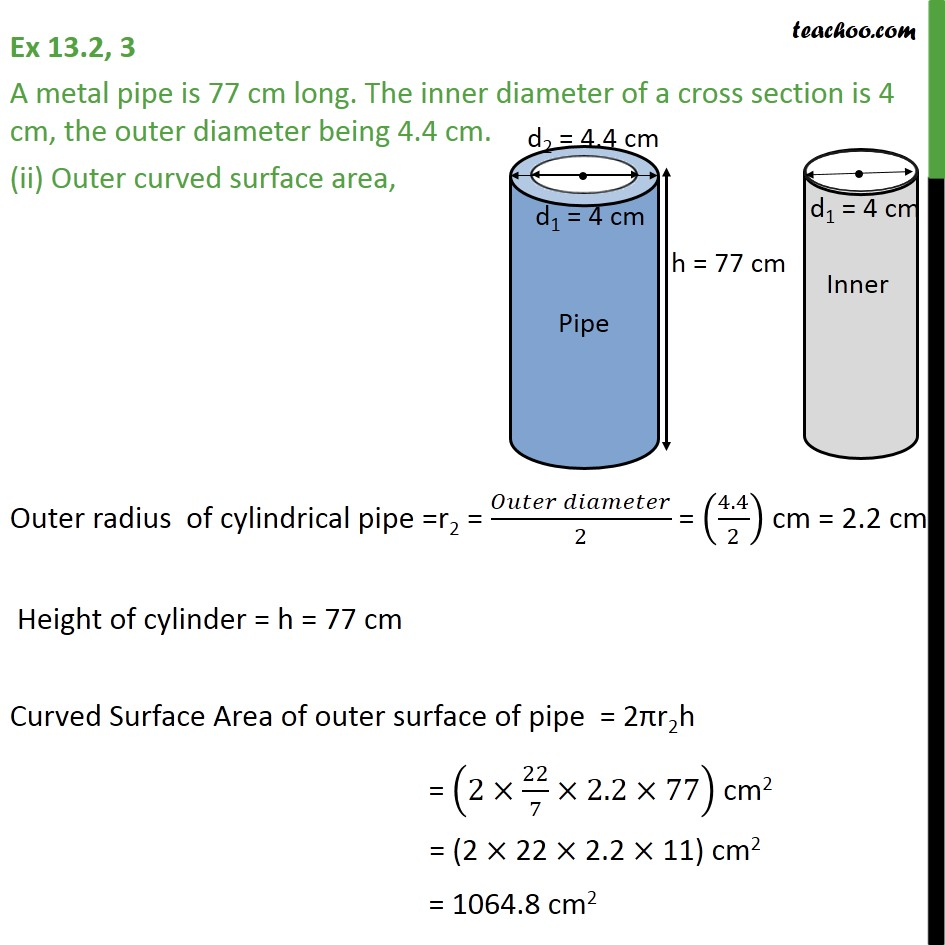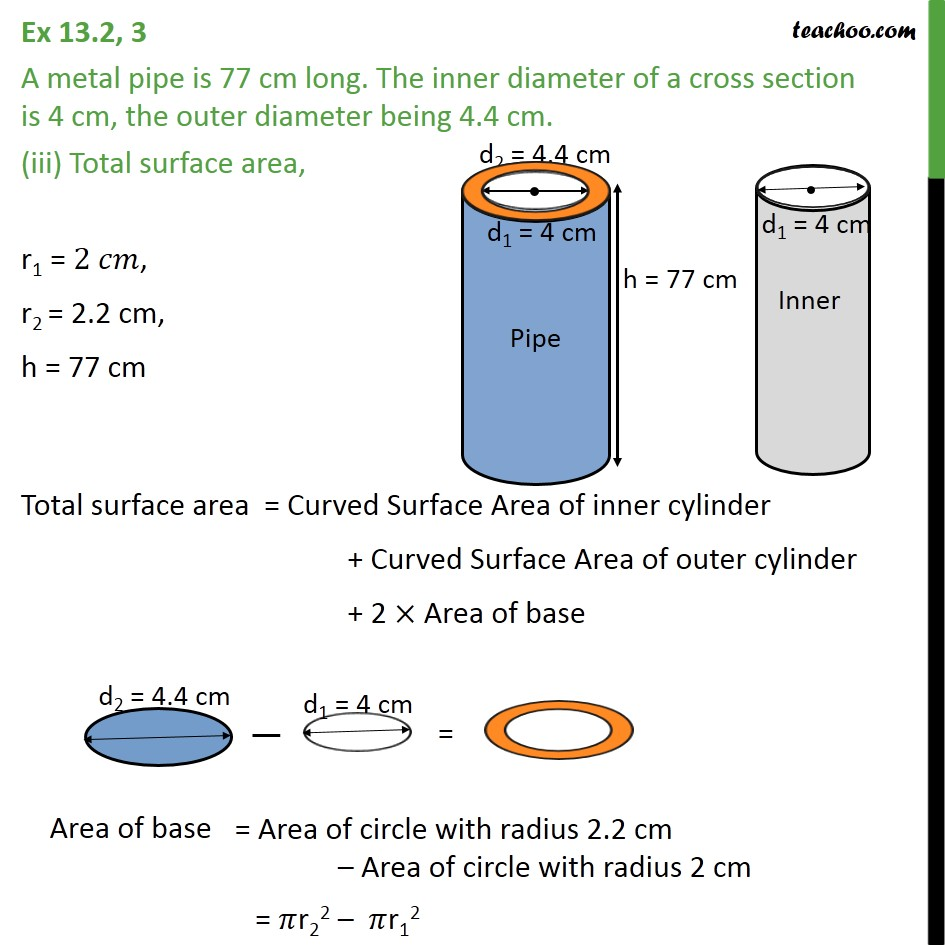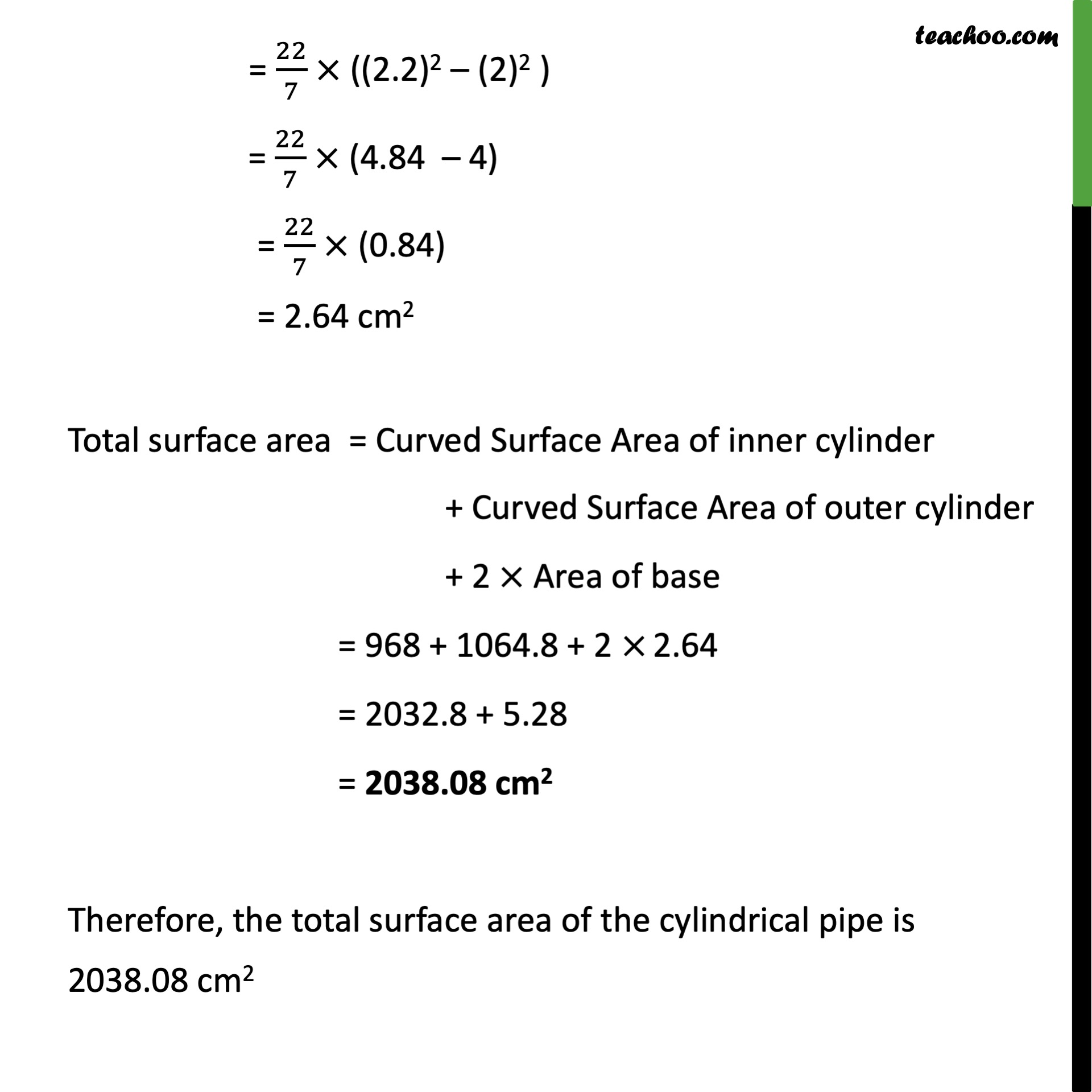Chapter 13 Class 9 Surface Areas and Volumes

Class 9
Important Questions for Exam - Class 9Learn in your speed, with individual attention - Teachoo Maths 1-on-1 Class

### Transcript

Question 3 A metal pipe is 77 cm long. The inner diameter of a cross section is 4 cm, the outer diameter being 4.4 cm. Inner curved surface area, Inner radius of cylindrical pipe = r1 = ( )/2 = (4/2) cm = 2 cm Height (h) of cylindrical pipe = 77 cm Curved Surface Area of inner surface of pipe = 2 r1h = (2 22/7 2 77) cm2 = 968 cm2 Question 3 A metal pipe is 77 cm long. The inner diameter of a cross section is 4 cm, the outer diameter being 4.4 cm. (ii) Outer curved surface area, Outer radius of cylindrical pipe =r2 = ( )/2 = (4.4/2) cm = 2.2 cm Height of cylinder = h = 77 cm Curved Surface Area of outer surface of pipe = 2 r2h = (2 22/7 2.2 77) cm2 = (2 22 2.2 11) cm2 = 1064.8 cm2 Question 3 A metal pipe is 77 cm long. The inner diameter of a cross section is 4 cm, the outer diameter being 4.4 cm. (iii) Total surface area, r1 = 2 , r2 = 2.2 cm, h = 77 cm Total surface area = Curved Surface Area of inner cylinder + Curved Surface Area of outer cylinder + 2 Area of base Area of base = r22 r12 = 22/7 ((2.2)2 (2)2 ) = 22/7 (4.84 4) = 22/7 (0.84) = 2.74 cm2 Total surface area = Curved Surface Area of inner cylinder + Curved Surface Area of outer cylinder + 2 Area of base = 968 + 1064.8 + 2 2.74 = 2032.8 + 5.76 = 2038.08 cm2 Therefore, the total surface area of the cylindrical pipe is 2038.08 cm2